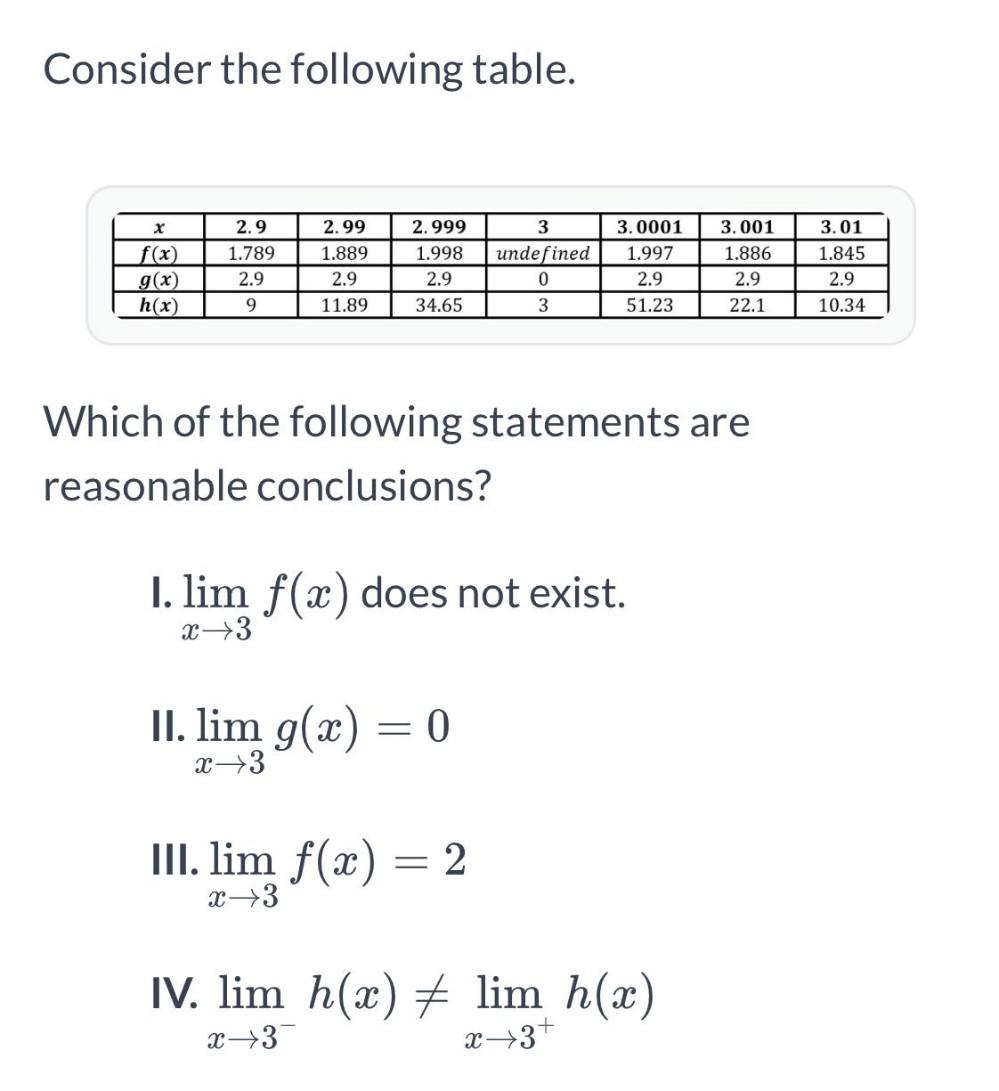Question:

# Consider the following table. 2.999 3.0001 2.9 1.789 f(x) g(x) h(x) 2.99 1.889 2.9 11.89 1.998 2.9 34.65 3 undefined 0 3. 001 1.Consider the following table. 2.999 3.0001 2.9 1.789 f(x) g(x) h(x) 2.99 1.889 2.9 11.89 1.998 2.9 34.65 3 undefined 0 3. 001 1.886 2.9 22.1 1.997 2.9 51.23 2.9 3.01 1.845 2.9 10.34 9 3 Which of the following statements are reasonable conclusions? I. lim f(x) does not exist. x 3 II. lim g(x) = 0 X X->3 III. lim f(x) = 2 = x +3 IV. lim h(x) # lim h(2) + x x3 x+3+